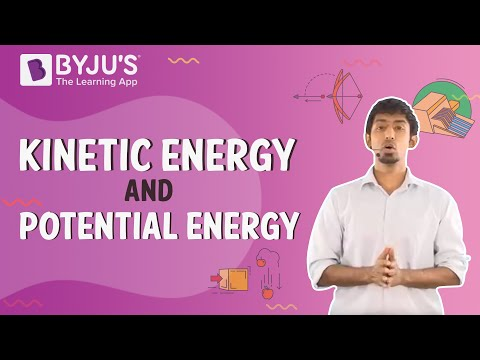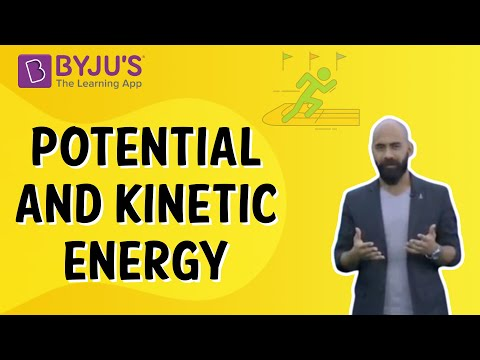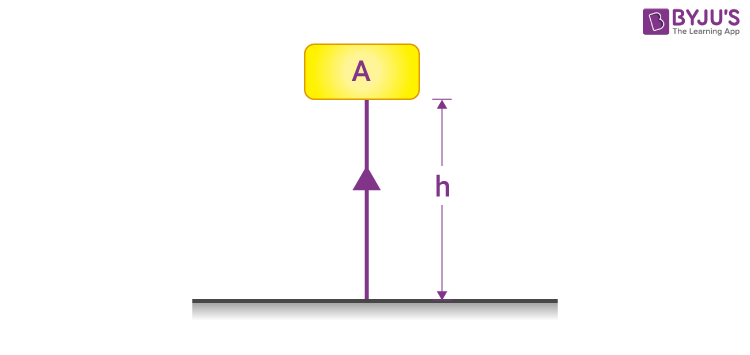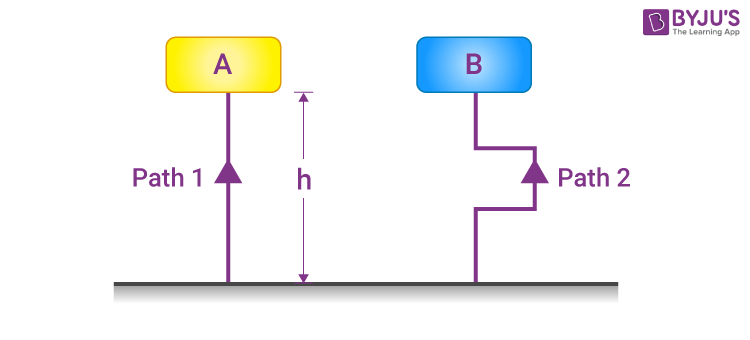# Potential Energy

The term potential energy was introduced by the 19th-century Scottish engineer and physicist William Rankine. There are several types of potential energy, each associated with a distinct type of force. It is the energy by an object’s position relative to other objects. In this article, let us learn what is potential energy, potential energy definition along with various examples and types.

## What is Potential Energy?

As we know, an object can store energy due to its position. In the case of a bow and an arrow, when the bow is drawn, it stores some amount of energy, which is responsible for the kinetic energy it gains when released.

Similarly, in the case of a spring, when it is displaced from its equilibrium position, it gains some amount of energy which we observe in the form of stress we feel in our hands upon stretching it. We can define potential energy as a form of energy that results from the alteration of its position or state.

After understanding potential energy and potential energy definition, let us learn the formula, unit, and examples of potential energy.

## Potential Energy Formula

The formula for potential energy depends on the force acting on the two objects. For the gravitational force, the formula is:

 W = m×g×h = mgh

Where,

• m is the mass in kilograms
• g is the acceleration due to gravity
• h is the height in meters

## Potential Energy Unit

Gravitational potential energy has the same units as kinetic energy: kg m2 / s2
Note: All energy has the same units – kg m2 / s2, and is measured using the unit Joule (J).

For more information on potential and kinetic energy, watch the below videos:You may want to check out the following related links

## Types of Potential Energy

Potential energy is one of the two main forms of energy. There are two main types of potential energy, and they are:

• Gravitational Potential Energy
• Elastic Potential Energy

### Gravitational Potential Energy

The gravitational potential energy of an object is defined as the energy possessed by an object that rose to a certain height against gravity. We shall formulate gravitational energy with the following example.

• Consider an object of mass = m.
• Placed at a height h from the ground, as shown in the figure.

As we know, the force required to raise the object equals m×g, that is, the object’s weight.As the object is raised against the force of gravity, some amount of work (W) is done on it.

Work done on the object = force × displacement.

So,

 W = m×g×h = mgh

Above is the potential energy formula.

As per the law of conservation of energy, since the work done on the object is equal to m×g×h, the energy gained by the object = m×g×h, which in this case is the potential energy E.

E of an object raised to a height h above the ground = m×g×hIt is important to note that the gravitational energy does not depend upon the distance travelled by the object, but the displacement, i.e., the difference between the initial and the final height of the object. Hence, the path along which the object has reached the height is not considered. In the example shown above, the gravitational potential energy for both blocks A and B will be the same.

## The below video provides Work and Energy quiz Class 9### Elastic Potential Energy

Elastic potential energy is stored in objects that can be compressed or stretched, such as rubber bands, trampolines and bungee cords. The more an object can stretch, the more elastic its potential energy is. Many objects are specifically designed to store elastic potential energy, such as the following:

• A twisted rubber band that powers a toy plane
• An archer’s stretched bow
• A bent diver’s board just before a diver dives in
• The coil spring of a wind-up clock

An object that stores elastic potential energy will typically have a high elastic limit. However, all elastic objects have a threshold to the load they can sustain. When deformed beyond the elastic limit, the object will no longer return to its original shape.

Elastic potential energy can be calculated using the following formula:

$$\begin{array}{l}U=\frac{1}{2}kx^2\end{array}$$

Where,

• U is the elastic potential energy
• k is the spring force constant
• x is the string stretch length in m

## Potential Energy Examples

### Potential Energy Practice Question:

Q1: What will be the gravitational potential energy of a ball of mass 1 kg when it is raised to 6 m above the ground. (g = 9.8 m s–2)

Solution:
Here, the mass of the object (m) = 1 kg,
Displacement (height) (h) = 10 m,
Acceleration due to gravity (g) = 9.8 m s–2.
Hence, Potential energy (P) = m×g×h = 1 kg × 9.8 m s–2 × 10 m = 98 J.

## Watch the video and solve NCERT exercise questions in the chapter Work and Energy Class 9## Frequently Asked Questions – FAQs

Q1

### Who coined the term potential energy?

The term potential energy was first used by a Scottish engineer and physicist named William Rankine during the 19th century. The concept of potential energy dates back to Aristotle.
Q2

### Name some objects that possess elastic potential energy.

Objects such as rubber bands, trampolines, and bungee cords all have elastic potential energy.
Q3

### What are the factors that gravitational potential energy an object depends on?

The amount of gravitational potential energy an object has depends on its height above the ground and its mass. The heavier an object and the higher it is above the ground, the more gravitational potential energy it has.
Q4

### What are the different types of potential energy?

The different types of potential energy are:

• Gravitational potential energy
• Elastic potential energy
• Chemical potential energy
• Electrical potential energy
• Nuclear potential energy
• Q5

### What is the relationship between potential and kinetic energy?

The relationship between potential and kinetic energy is that they can be transformed into each other. This can be explained by considering the water stored in a tank. When the tap of the tank is closed, water in the tank possesses potential energy. If the tank’s tap is opened, water will possess kinetic energy.

## Watch the video and solve complete exemplar questions in the chapter Work and Energy Class 9Hope you understand in detail what potential energy is and what potential energy definition, examples, and types are. Stay tuned to BYJU’S – The Learning App to learn about various science and maths concepts.

Test your Knowledge on Potential Energy